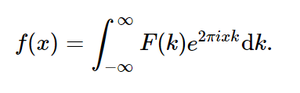# sympy.integrals.transforms.inverse_fourier_transform() in python

With the help of inverse_fourier_transform() method, we can compute the inverse fourier transformation and return the unevaluated function.Inverse Fourier Transformation

Syntax :  inverse_fourier_transform(F, k, x, **hints)

Return : Return the unevaluated function.

Example #1 :

In this example we can see that by using inverse_fourier_transform() method, we are able to compute the inverse fourier transformation which return the unevaluated function by using this method.

## Python3

 `# import inverse_fourier_transform ` `from` `sympy ``import` `inverse_fourier_transform, exp, sqrt, pi ` `from` `sympy.abc ``import` `x, k ` ` `  `# Using inverse_fourier_transform() ` `gfg ``=` `inverse_fourier_transform(sqrt(pi)``*``exp(``-``(pi ``*` `k)``*``*``2``), k, x) ` ` `  `print``(gfg)`

Output :

exp(-x**2)

Example #2 :

## Python3

 `# import inverse_fourier_transform ` `from` `sympy ``import` `inverse_fourier_transform, exp, sqrt, pi ` `from` `sympy.abc ``import` `x, k ` ` `  `# Using inverse_fourier_transform() ` `gfg ``=` `inverse_fourier_transform(sqrt(pi)``*``exp(``-``(pi ``*` `k)``*``*``2``), k, ``4``) ` ` `  `print``(gfg)`

Output :

exp(-16)

My Personal Notes arrow_drop_upCheck out this Author's contributed articles.

If you like GeeksforGeeks and would like to contribute, you can also write an article using contribute.geeksforgeeks.org or mail your article to contribute@geeksforgeeks.org. See your article appearing on the GeeksforGeeks main page and help other Geeks.

Please Improve this article if you find anything incorrect by clicking on the "Improve Article" button below.

Article Tags :

Be the First to upvote.

Please write to us at contribute@geeksforgeeks.org to report any issue with the above content.